Book: DiscoveryInMathematics

See: Math discovery

Discovery in Mathematics: A System of Deep Structure

I will talk about how we may systematically study the ways of figuring things out in mathematics.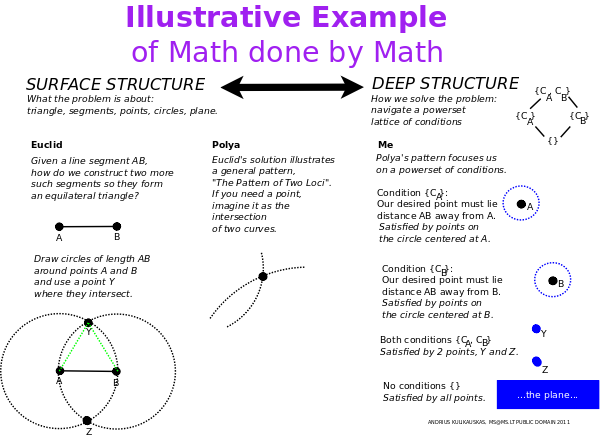George Polya, in his book, "How to Solve It", considers Euclid's problem of how to construct an equilateral triangle. If we are given the side AB, how do we construct the other two? The solution is a recurring idea which Polya calls the "pattern of two loci". We think of there being two separate conditions. One side must extend a length AB from the point A. Another side must extend a length AB from the point B. We thus draw two circles of radius AB centered at A and B. The points where the two circles intersect are those where we can draw a third point C which satisfies both conditions so that our triangle is equilateral.

I realized that our minds solve this problem by imagining a powerset lattice of conditions. Circle A is one condition, circle B is another condition, and the intersection of A and B satisifies the union of these two conditions. Our minds have thus solved the surface problem (constructing a triangle) by considering a simpler, deeper structure (a lattice of conditions). This brings to mind linguist Noah Chomsky's work in syntax and architect Christopher Alexander's work on pattern languages.

I collected such problem solving patterns discussed in Paul Zeitz's book The Art and Craft of Problem Solving and other sources. Each distinct pattern makes use of a structure which is familiar to mathematicians and yet is not explicit but mental. We may consider those math structures to be cognitively "natural" which are used by the mind in solving math problems. I present to you 24 patterns which I identified and systematized them in a way which suggests they are complete.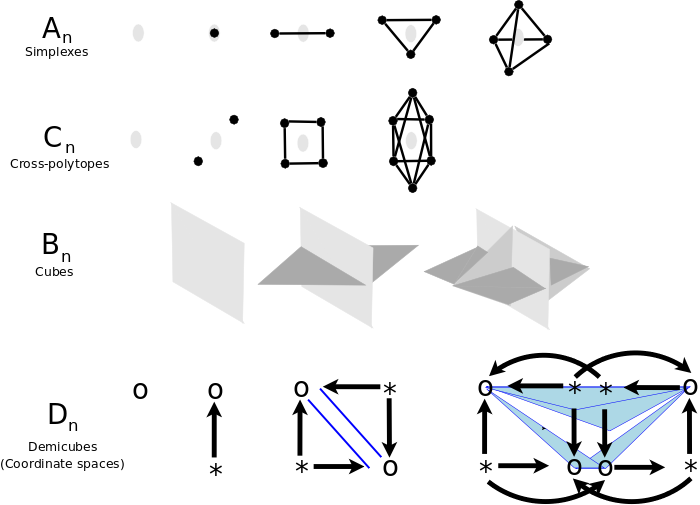Let me first show how to systematically generate the 4 infinite families of polytopes, An (simplexes), Cn (cross polytopes), Bn (hypercubes), Dn (demicubes), whose symmetry groups are also the Weyl groups for the root systems of the classical Lie algebras. The resulting structures, actions and relationships will help me talk systematically about the mathematical ways of figuring things out.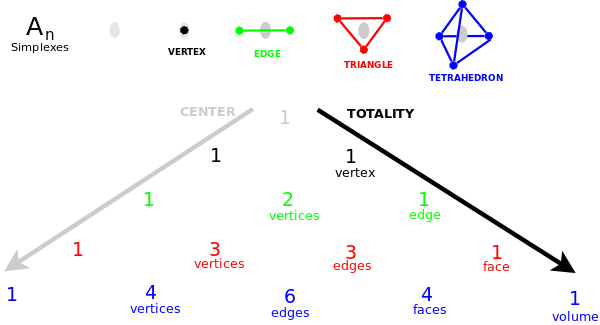I define the Center of a polytope as that which is not a vertex but which ever generates the next vertex by which the polytope may grow. I interpret it as the unique center of the polytope which keeps moving as the polytope grows. For example, the simplexes An are generated by adding one vertex at a time, along with edges to all of the existing vertices, which makes clear that it is indeed a new vertex. The k-dimensional subsimplexes of the n-dimensional simplex are given by the n+1st row of Pascal's triangle. I interpret the Center to be the -1 simplex, the unique leftmost sub-simplex in each row which has no vertices and is -1 dimensional. Note that the dual of the Center is the Totality, the unique rightmost sub-simplex in each row which has all n vertices.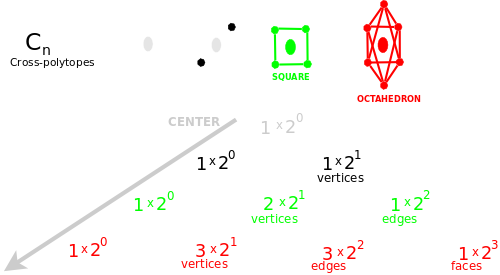The cross polytopes Cn are generated as follows. The Center generates two vertices at a time, not connected to each other, yet connected to all other vertices. Thus B1 is two vertices, B2 is a square and B3 is an octahedron. The symmetry group not only permutes the vertices, but also reflects opposite vertices in each direction. Each dimension may be thought of independently as modeling opposite qualities. If we look at the relevant combinatorial triangle we conclude that Cn has a Center but no Totality. For example, the rightmost entry for the octahedron is given by its 8 faces of 3 vertices each. In this sense, the octahedron is 3-dimensional but it has no volume.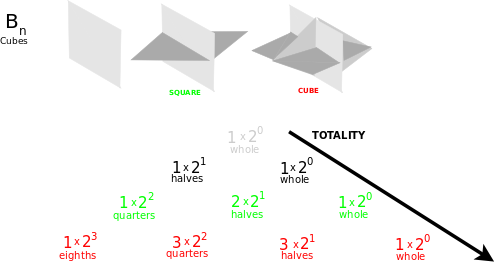The cubes Bn are a dual interpretation of the same combinatorial triangle as for the Cn. They have a Totality but no Center. We may think of the Totality as generating an unfolding sequence of perpendicular mirrors. These mirrors define quadrants, fractions of the whole, which are ever divided anew. Each mirror defines an opposition and each quadrant is distinguished by the entire sequence of oppositions.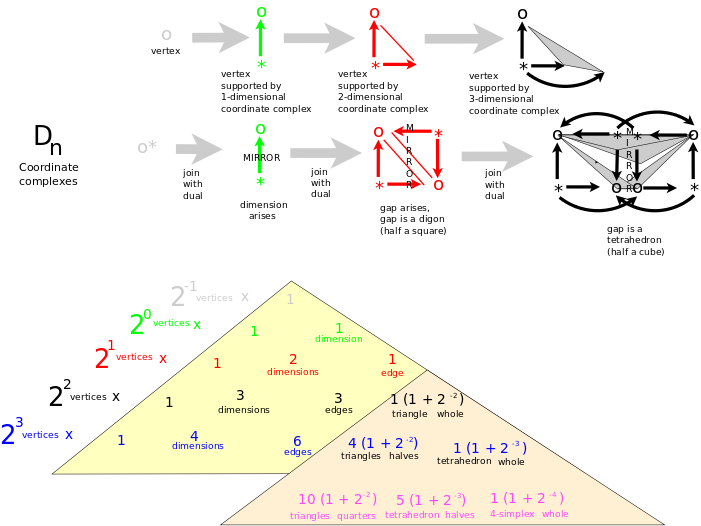We may think of the demicubes Dn as having no Center and no Totality. Typically, a demicube is defined by starting with a cube and retaining and discarding alternate vertices. Instead, let us choose any vertex (a quadrant) and make it an Origin, an Anti-Center, by folding the cube in half, that is, fusing to it the vertex which is opposite to it in every way, and likewise turning and fusing pairs of vertices to create a coordinate system of unit vectors around that Origin. Link with edges all of the tips of the unit vectors to get a simplex. We can think of each such coordinate system as serving one of the vertices. The coordinate systems fit together (their edges overlap) to bound the demicube. We can think of the coordinate systems as forming a coordinate complex which fosters duality and ambiguity. There are two different ways in which we can think of the coordinate complex as growing at each step. On the one hand, we can think of each coordinate system as growing like a simplex but with a distinguished Origin and unit vectors to the other vertices. On the other hand, we can think of each dimension arising by introducing a mirror which reflects a diagram into its dual (in which Origins are exchanged with Vertices and vectors reversed) and joins it with that dual. Thus vectors lead out from the Origins to the Vertices and the Vertices are linked by pairs of edges. Also, a gap opens up between the coordinate systems. This gap bounds the demicube and its parts, which are halves, quarters, eighths and so on, with the smallest part being the tetrahedron. These sequences of parts gives us a top-down point of view onto specific coordinate systems, whereas each coordinate systems allow us to construct a bottom-up point of view. Thus we may imagine that the coordinate complex has no Center, but rather it has a multitude of Origins, each of which loves some vertex with a coordinate system. And the coordinate complex has no Totality of vertices, but rather it defines a whole that is the gap between the coordinate complexes.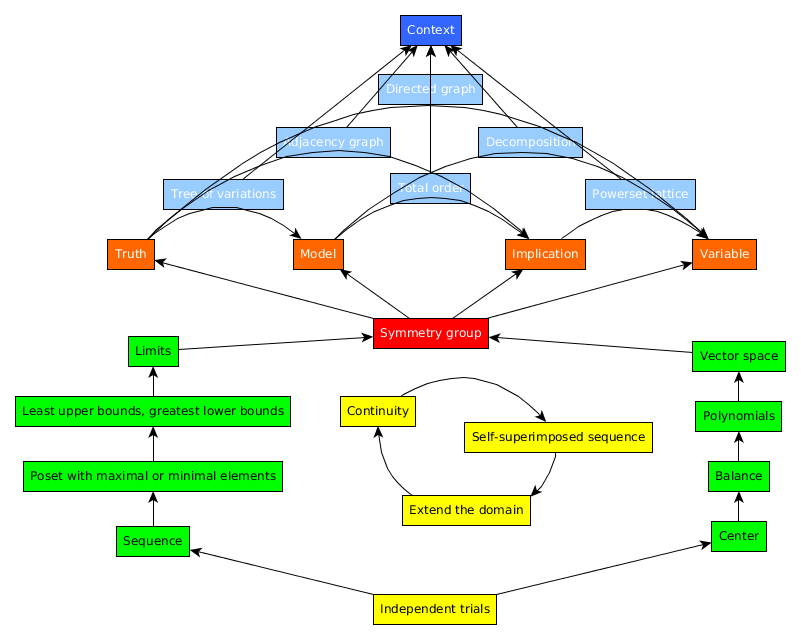We can now imagine how the polytopes An, Cn, Bn and Dn are the foundations for 4 different kinds of geometries. Indeed, these geometries are the basis for 4 different ways of metalogically figuring things out.

The simplexes An span all of space from the Center and so are the basis for affine geometry where directions are preserved. We figure things out metalogically by preserving the relationship between a metalogical perspective of the Center outside of a system and the logical perspective of a vertex inside a system. Metalogically, the simplexes let us figure out whether things are true by leveraging the inherent contradiction within the Center, the unresolvable tension between its form and content, which allows us to view two options and yet deem one true by contradiction. Simplexes model a theology where "God is good" in every direction.

The cross-polytopes Cn define opposite directions and so are the basis for projective geometry where lines are preserved. Objects are constructed from independent dimensions which is key for algebraic ways of figuring things out. Metalogically, we figure out what is true by simplifying, even by "wishful thinking", modeling the most relevant dimensions. Cross-polytopes model a theology where every dimension represents a choice, potentially good or bad.

The cubes Bn relate perpendicular directions and so are the basis for conformal geometry where angles are preserved. Manifolds are freed within multidimensional spaces which is key for analytic ways of figuring thing out. Metalogically, we figure out how things are true by working backwards from a multidimensional point to the spaces which are its conditions. Cubes model a theology where every quadrant is defined by a collection of choices made.

The coordinate systems Dn superimpose directions and angles and so are the basis for symplectic geometry where areas are preserved. We figure things out logically by applying transformations to bridge the gap between the perspectives given by a vertex in a system and a vertex in a subsystem. Metalogically, we figure out why things are true by interpreting variables in ways that clarify the semiotic disconnects between these four geometries. Our minds distinguish semiotic levels of thing (whether), icon (what), index (how), symbol (why), as when we draw a picture. We use variables to imagine a broader level as free but a narrower level as set. We thus interpret variables as dependent vs. independent, known vs. unknown, given vs. arbitrary, fixed vs. varying, concrete vs. abstract, defined vs. undefined and so on. Coordinate systems model a theology where good is distinguished from bad and yet truly the system is self-centered, relative and questionable. Consequently, "God does not have to be good", life does not have to be fair.

We can think of each of these four geometries as relating the explicit math on a sheet of paper and the implicit math by which we interpret it with our minds. One way to figure things out is to realize that we can always start with a fresh sheet. We can run independent trials, vary them, get our hands dirty, accumulate some data points, add some noise, restate what we have formulated, start all over again. We are thus starting without any system, but our goal is a second way of figuring things out, namely to structure our sheet as a completely defined system which our mind can play with, acting on it like a symmetry group, constructing strings of actions and undoing them at will, applying mental functions and their inverses, as we wish. Yet ultimately there is a third way by which we figure out the limits of our knowledge. If I ask you, what is 10+4? You may say 14, but the answer is 2, because I am thinking about a clock. Which is to say that what we know may be completely irrelevant and plain wrong because ultimately it all depends on context. We have to be willing to go back to the blank sheet.

Even so, we seek a symmetric group which brings together algebraic structures and analytic dynamics. The four polytopes An, Cn, Bn, Dn illustrate the ways that structure defines helpful perspectives. We can solve a problem by recognizing the center of the whole, as suggested by the simplex. Or we can make use of parity as suggested by the cross-polytopes. We do this when we modify an equation with terms that add to zero or that multiply to one, or when we consider a subset and its complement, whereby elements only differ as to whether they are in or out of a set. Yet there is another way of looking at a set such that its elements are all distinct, as when we combine a variety of amounts and units, as suggested by the cubes. Finally, we can construct or deconstruct a vector subspace in an orderly manner given a list of canonical basis elements, as suggested by the coordinate systems.

The four polytopes likewise inspire dynamic analytic perspectives, though not within a single sheet, but through a sequence of sheets. We can apply mathematical induction, as suggested by the center of a simplex, in that it ever generates the next vertex. We can imagine a maximum and minimum in any dimension, as suggested by the cross-polytopes. The quadrants of the cubes help us imagine that various solutions can serve as least upper bounds or greatest lower bounds. And the twofold nature of the coordinate systems Dn let us imagine that if we generate a sequence of vertices, then it will ultimately achieve its limit somewhere in the half cube.

We also imagine functors which couple the algebraic structural and analytical dynamic points of view through the scientific method of taking a stand (hypothesizing), following through (experimenting) and reflecting (concluding). We imagine this coupling ambiguously as both strict and loose, so that slack can decrease but also increase. Given a constraint such as the addition formula (2**X)(2**Y) = 2**(X+Y), we take a stand by extending the domain, stitching together new values for X and Y, such as zero, negative integers, fractions, real numbers, complex numbers and so on. Next, we can experiment in our minds, varying these variables continuously, seeing whether our model will break or hold. Finally, we can generalize and reformulate what we have learned by considering our constraint as a recurrence relation and superimposing the resulting sequence upon itself, as with a generating function, or as with auto-associative memory, where time-delay lets patterns be related to themselves. This three-cycle of extending the domain, varying continuously and self-superimposing is what I imagine brings forth the particular structures and actions which our mind systematizes with some symmetry group.

Once we have established our symmetry group and a comprehensive system, then our four geometries let us analyze it theologically by imagining how the Center outside relates invariantly to the vertices inside. But we can also analyze our system ethically by considering how a transformation links the perspective of a vertex within a system with the perspective of a vertex within a subsystem. Six transformations bridge the gap between one geometry's analytic dynamics and another geometry's algebraic structure. We can illustrate these transformations by considering the different senses that we ascribe to symmetry group actions, but in particular, to multiplication. In each case, the transformation restructures structure of one kind with a structure of another kind. We find natural grounds for six of the axioms of Zermelo-Fraenkel set theory.

We can imagine people in a system as vertices with perspectives, that is, with edges, with relationships to other people, vertices, perspectives. Let us think of love as the loose coupling which identifies a perspective as the same perspective after a three-cycle of extending the domain, varying continuity and self-superimposing. We can imagine that structurally a domain extends like a tree, continuity asserts itself as a sequence, and self-superimposition imposes itself as a network. Symmetry is that equivalence which allows us to imagine vertices as different and yet treat them as the same. Structurally, symmetry can ground an equivalence at various levels of granularity: the whole, as characterized by the unity of the center; multiples, as given by what is the same and not different; and the set, where elements are labeled but not ordered. Whereas a list allows no symmetry. Dynamically, we must operate at a finer granularity. If the world is a list of items, then we help our loved one love all by redistributing it. If people are elements in a set, then we help our loved one rescale their relationships so they love each other. Inasmuch as our loved one is an ideal person, then we help them love themselves by reaffirming, recopying themselves. Thus we love them as we love ourselves. In these ways, symmetry loosely couples structure and dynamics to define transformations which bridge perspectives through loving equivalences.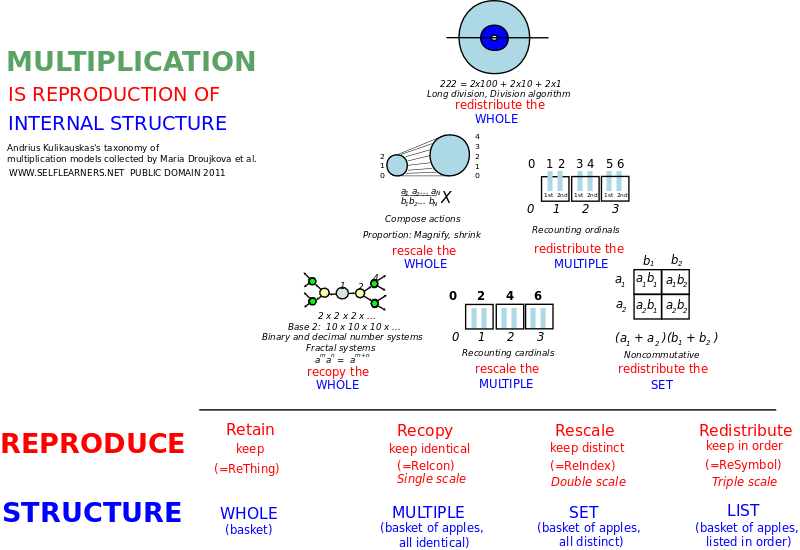In figuring things out logically within a well defined mathematical system, our mind grants the slack by way of six different transformations, each of which is familiar from multiplication. For example, a tree of variations can be restructured by a sequence to visualize evolution. Our mind visualizes fractal self-similarities and, as in multiplying powers of two, recopies the whole in a self-love. Such gathering of branches grounds the Axiom of Pairing in set theory.

A network of relationships is restructured by a tree. We visualize this as an atlas of global and local views upon adjacencies. Similarly, multiplication composes actions to rescale the whole, to magnify or shrink relationships, and love reciprocally. The Axiom of Extensionality states that sets are globally equal if and only if their elements are locally equal.

A sequence of instructions is restructured by a network of redirects to visualize a handbook. Analogously, multiplication rescales multiples by tallying and skip counting. It leverages the total order in order to recount cardinals. This grounds the Well-ordering Theorem and the Axiom of Choice.

We can consider the dual of our three-cycle, the conditions which allow us to take a stand, follow through, and reflect. We thus accept our world in its finest granularity to allow for that redistribution of truth by which we might all love unconditionally in total harmony. A sequence of events is restructured by a tree of possibly overlapping time periods. We thus visualize a chronicle. Multiplication redistributes a set as with box multiplication and lets us disentangle "ands" and "ors". Thus in our minds we organize a power set lattice of conditions. This grounds the Axiom of Power Set.

However, our redistribution need not be so completely refined. A tree of concepts is restructured by a network of partial references to visualize a catalog. Multiplication lets us redistribute multiples by factoring components, thus regrouping and recounting ordinals. This grounds the Axiom of Union.

Our redistribution may apply to a whole, as with long division, where we keep having a remainder. A network of causes and effects is restructured by a sequence to visualize a tour. Our minds analyze the path to consider, especially, whether it will avoid cycles, or whether they will be stable or unstable. This grounds the Axiom of Regularity.

Inherent in each of these six transformations is a slack in the system, which taken together, maintains that we be obedient to the possibility that everything we know is wrong.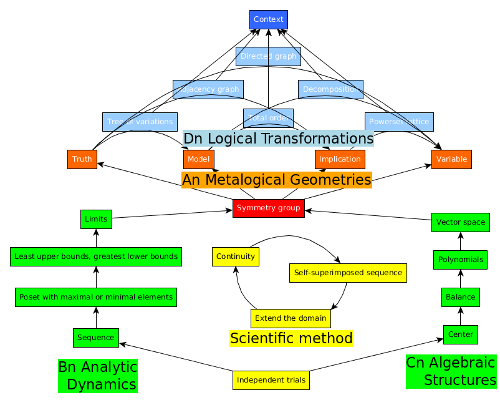Overviewing this entire system, I imagine it as spawned by the inner tension of the Center, which is first characterized by the ambiguity of a symmetry group's action and structure. This tension then divides itself for the duality of the Center's blank sheet from which we start and the Totality's total context which we may yet need to completely reinterpret. Then the internal slack of the symmetry group is made concrete through the loose coupling of that three-cycle of extending the domain, varying continuously and self-superimposing, which allows for equivalences. Finally, the primacy of the Center in its duality with the Totality is evinced by the four polytope families, whereby the duality of algebraic structure (constructed bottom-up by the cross-polytopes Cn) and analytical dynamics (unleashed top-down by the cubes Bn) is coupled by the three-cycle to ground a self-standing system around a symmetry group, defined metalogically (by the simplexes An) to express logical slack (through the coordinate systems Dn) which establishes the absolute vulnerability of the Totality and thus the Center's primacy and necessary reality.

I am deeply grateful to Maria Droujkova and the Natural Math team for investigating the many senses of multiplication, and to Kirby Urner for pointing out the differences in tetrahedral and cubic thinking, in the spirit of Buckminster Fuller. Thank you also to Thomas Gajdosik for his supportive understanding in our many conversations.

Parsiųstas iš http://www.ms.lt/sodas/Book/DiscoveryInMathematics
Puslapis paskutinį kartą pakeistas 2018 lapkričio 11 d., 16:38